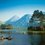# OPC 2 Problem 5

$5. \text{Prove that if } n \ge 4 \quad then \quad n , n+2,n+4 \text{ can't all be primes.}$

Give different methods of solving this. This was a problem to the OPC 2. The OPC 2 has already ended. OPC 3 will be released soon.Calvin sir suggested me to do this.So Thanks to him.$\ddot\smile$Note by Rajdeep Dhingra
6 years, 4 months ago

This discussion board is a place to discuss our Daily Challenges and the math and science related to those challenges. Explanations are more than just a solution — they should explain the steps and thinking strategies that you used to obtain the solution. Comments should further the discussion of math and science.

When posting on Brilliant:

• Use the emojis to react to an explanation, whether you're congratulating a job well done , or just really confused .
• Ask specific questions about the challenge or the steps in somebody's explanation. Well-posed questions can add a lot to the discussion, but posting "I don't understand!" doesn't help anyone.
• Try to contribute something new to the discussion, whether it is an extension, generalization or other idea related to the challenge.

MarkdownAppears as
*italics* or _italics_ italics
**bold** or __bold__ bold
- bulleted- list
• bulleted
• list
1. numbered2. list
1. numbered
2. list
Note: you must add a full line of space before and after lists for them to show up correctly
paragraph 1paragraph 2

paragraph 1

paragraph 2

[example link](https://brilliant.org)example link
> This is a quote
This is a quote
    # I indented these lines
# 4 spaces, and now they show
# up as a code block.

print "hello world"
# I indented these lines
# 4 spaces, and now they show
# up as a code block.

print "hello world"
MathAppears as
Remember to wrap math in $$ ... $$ or $ ... $ to ensure proper formatting.
2 \times 3 $2 \times 3$
2^{34} $2^{34}$
a_{i-1} $a_{i-1}$
\frac{2}{3} $\frac{2}{3}$
\sqrt{2} $\sqrt{2}$
\sum_{i=1}^3 $\sum_{i=1}^3$
\sin \theta $\sin \theta$
\boxed{123} $\boxed{123}$

Sort by:

• Statement1:- A prime number greater than 2 must be odd

• Statement 2 : - A prime number n when divided by any number d , such that d < n , we get a remainder of either 1 or 2 .

Restating the above statement: .... If

(n =Prime Dividend, q = Quotient, and d = Divisor ) , given 0<d<n

   1.  n = d x q

2. n=d x  q  + 1

3. n= dx q +2


Now , for n>= 4 , let d=3
then q must be greater than or at least equals to 1 , ie. q>= 1

case 1: For q > 1

   a) n= 3 x q        {Not Prime}

b)   n=3x q  +1 ....

or,    n+2=3 x (q+1)     { Not Prime}

c) n=3x q  +2

or , n+4=3x  (q+2)      { Not Prime}


so we can see that ( n , n+2 and n+4 ) cannot be all primes for each a) , b) and c) since they are product of 3 and a positive integer

case 2 :For q = 1

   a) n=4

b) n=5


.for n=4 , n+2 and n+4 are not primes , also for =5 , n+4 is not prime .

Hence we can assert that for n>=4 n, n+2, n+4 cannot be all Primes

- 6 years, 4 months ago

Let n be prime, so n is odd.

Case1: n mod 5=1, then n+4 mod 5 =0, so n+4 is not prime. Case2: n mod 5=3, then n+2 mod 5 =0, so n+2 is not prime.

Complete!

- 6 years, 4 months ago

one of them will be divisible by 3. so they cant all be primes

- 5 years, 6 months ago

Not necessarily..

- 5 years, 3 months ago

Sorry That Was A typo.The

real statement should be one of them will be divisible by 3 so they cant all be primes

- 5 years, 3 months ago

Hm, thats correct now.

- 5 years, 3 months ago